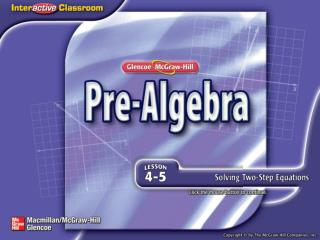DownloadDownload PresentationSplash Screen

# Splash Screen

Télécharger la présentation## Splash Screen

- - - - - - - - - - - - - - - - - - - - - - - - - - - E N D - - - - - - - - - - - - - - - - - - - - - - - - - - -
##### Presentation Transcript

1. Splash Screen

2. Then/Now New Vocabulary Example 1: Solve a Two-Step Equation Example 2: Solve a Two-Step Equation Example 3: Equations with Negative Coefficients Example 4: Combine Like Terms Before Solving Example 5: Standardized Test Example Lesson Menu

3. You have already solved one-step equations. (Lessons 4–3 and 4–4) • Solve two-step equations. • Solve real-world problems involving two-step equations. Then/Now

4. two-step equation Vocabulary

5. Solve 2m + 8 =32. Check your solution. – 8 = – 8 Subtraction Property of Equality Division Property of Equality Solve a Two-Step Equation Method 1 The Vertical Method 2m + 8 = 32 Write the equation. 2m = 24 Simplify. m = 12 Simplify. Example 1

6. Division Property of Equality Solve a Two-Step Equation Method 2 The Horizontal Method 2m + 8 = 32 Write the equation. 2m + 8 – 8 = 32 – 8 Subtraction Property of Equality 2m = 24 Simplify. m = 12 Simplify. Answer: The solution is 12. Example 1

7. Solve 5b + 4 = 79. A. –70 B. –15 C. 15 D. 70 Example 1

8. Write the equation. Subtraction Property of Equality Simplify. Multiplication Property of Equality Solve a Two-Step Equation w = 12 Simplify. Answer: The solution is 12. Example 2

9. A. 1 B. 6 C. 12 D. 30 Example 2

10. Solve 5 – x =7. Divide each side by –1. Equations with Negative Coefficients 5 – x = 7 Write the equation. 5 – 1x= 7 Identity Property; x = 1x 5 + (–1x) = 7 Definition of subtraction –5 + 5 + (–1x) = –5 + 7 Add –5 to each side. –1x = 2 Simplify. x = –2Simplify. Answer: The solution is –2. Example 3

11. Solve 9 = –4 – m. A. 13 B. 5 C. –5 D. –13 Example 3

12. Solve b – 3b + 8=18. Check your solution. Divide each side by –2. Combine Like Terms Before Solving b – 3b + 8 = 18 Write the equation. 1b – 3b + 8 = 18 Identity Property; b = 1b –2b+ 8 = 18 Combine like terms, 1b and –3b. –2b + 8 – 8 = 18 – 8 Subtract 8 from each side. –2b = 10 Simplify. b = –5Simplify. Answer: The solution is –5. Example 4

13. A.1 B. C. D.–1 Solve 9 = 13 – x + 5x. Example 4

14. Marissa wants to go to summer camp. The camp costs \$229. She paid a deposit of \$75, and she will need to save \$14 per week to pay for the trip. The equation 75 + 14w = 229 can be used to find how many weeks Marissa will need to save. Which series of steps can be used to solve the equation? A Divide 229 by 14. Then subtract 75. B Subtract 14 from 229. Then divide by 75. C Subtract 75 from 229. Then divide by 14. D Subtract 229 from 75. Then divide by 75. Example 5

15. Division Property of Equality Read the Test ItemSolve the equation so that you can write the steps in the correct order that are necessary to solve the problem. Solve the Test Item 75 + 14w = 229 Write the equation. 75– 75 + 14w= 229 – 75 Subtraction Property of Equality 14w = 154 Simplify. w = 11 Simplify. Example 5

16. Check Answer: To solve the equation, you first subtract 75 and then divide by 14. The correct answer is C. When you solve an equation, you undo the steps in evaluating an expression in reverse order of the order of operations. In this equation, you would first undo adding 75 by subtracting 75, then undo multiplying by 14 by dividing by 14. Example 5

17. Adrienne is saving to buy a new MP3 player that costs \$125. She has a summer job where she earns \$6 per hour. She already has \$17. The equation 17 + 6h = 125 can be used to find how many hours Marissa will need to work to pay for her player. Which series of steps can be used to solve the equation? A. Divide 125 by 6. Then subtract 17. B. Subtract 6 from 125. Then divide by 17. C. Subtract 17 from 125. Then divide by 6. D. Subtract 125 from 17. Then divide by 17. Example 5

18. Click the links below to watch video examples of 2-step equation problems. Be sure to check your assignments on ConnectEd. • Video 1 • Video 2 • Video 3 With Online Practice • Video 4 • Video 5

19. End of the Lesson